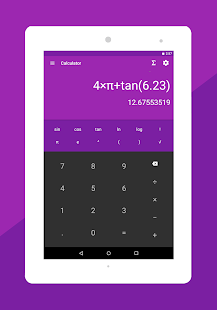Scientific calculator with maths formulas is a best education tool. There are more than 1000 formulas organised neatly. The In-Built calculator has both scientific mode and standard mode. The app also features built in maths related Applications and converters.

Best Features of the App
⋆ Calculator ⋆
• Basic Calculations like addition, subtraction, multiplication and division.
• Advanced scientific calculations like logarithmic, trigonometric, and exponential functions
• comes with Dark theme and light theme with various colours.
• chose between radian and degrees

⋆ Maths Formulas ⋆
Algebra : Factoring, Roots, Powers, Complex Numbers and Equations.
Geometry : Cone, Cylinder, Square, Sphere, Rectangle, Triangle, Trapezoid. Etc…
Analytical Geometry : Circle, Hyperbola, Ellipse, Parabola etc…
Integration Functions : Properties of Integration, rational functions, Trigonometric Functions, Hyperbolic functions, Exponential and log functions
Derivations : Properties of derivation, general derivative, hyperbolic and inverse hyperbolic functions etc…
Trigonometry : Sine and cosine Rule, Table of angle, Angle transformations, Euler’s formula etc…
Laplace Transformation : Properties and Functions of Laplace transformation
Numerical Methods : Roots of Equation, Numerical Integration, Lagrange and Newton’s Interpolation
Probability : Expectation, Variance, Distribution, Permutation and Combination etc…
Fourier Series : Fourier transform operations and Table of Fourier Transform
Series : Arithmetic Series, Geometric Series, Finite and Binomial Series.
• Formulas Search Functionality
Matrices : Transpose, Addition, Multiplication, Inverse of a Matrix
Equations : Linear, Quadratic, Exponential, Log Equations and InEquations
• Surface Area, 3D – Geometry, Coordinated Geometry, Logarithms, Circles, Planes

⋆ Maths Applications ⋆
• Number Base Converter : Binary, Decimal, Octal and HexaDecimal Converters
• Number Series Generator : Arithmetic Series, Geometric Series, Fibonacci Series
• Volume Calculation : Volume of Cone, Cylinder, Rectangle, Circle, Tent and Trapezoid
• Area Calculation : Area of Circle, Rectangle, Triangle and Trapezoid.
• Roman Numerals : Decimal Number to Roman Numeral Converter.
• Ratio Calculator
• Proportion Calculator
• Decimal to Fraction Converter
• GCD and LCM calculator
• Random Number Generator

P.S Maths Formulas with Calculator is created proactively with wide range of users from Students, Engineer and Professionals in mind. This app is a irreplaceable daily utility for those who find it difficult to keep track of all important maths and Engineering Formulas. The in-built Scientific Calculator and Maths Applications and Converter is a added bonus feature of the App. Thanks for your continuous Support. We have lot more formulas and features coming up in the future updates.

WHAT’S NEW

✓ 1000+ important Maths Formulas
✓ Chemistry Formulas
✓ Logarithm, Surface Area, Circles, Planes and more new categories
✓ Maths Applications and Calculator
✓ Fixes

Screenshots

•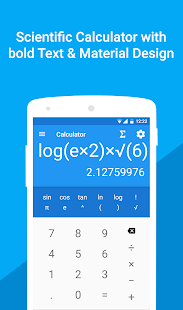•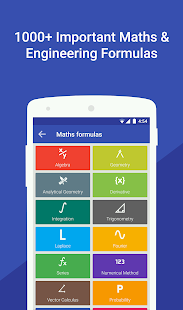•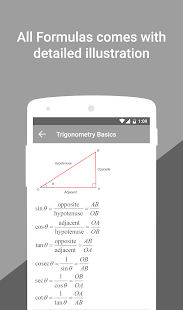•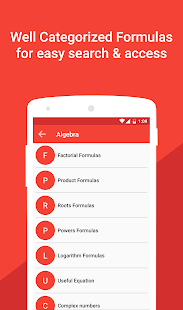•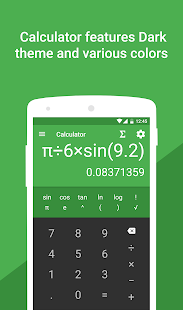•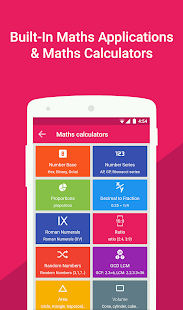•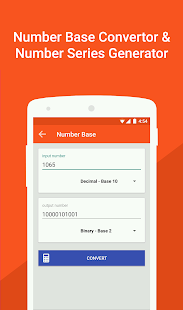•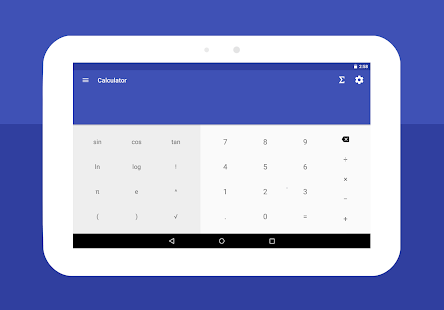•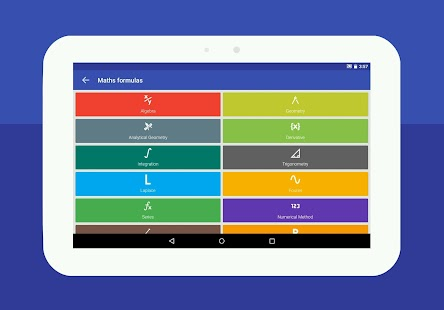••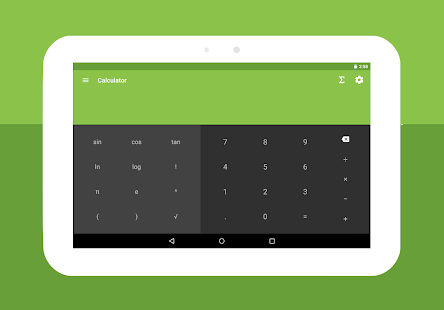•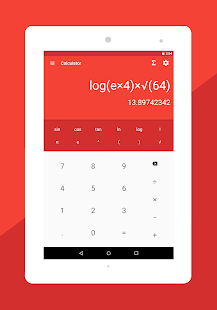•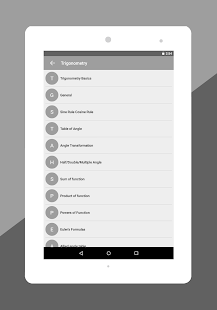•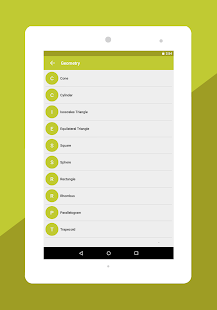•